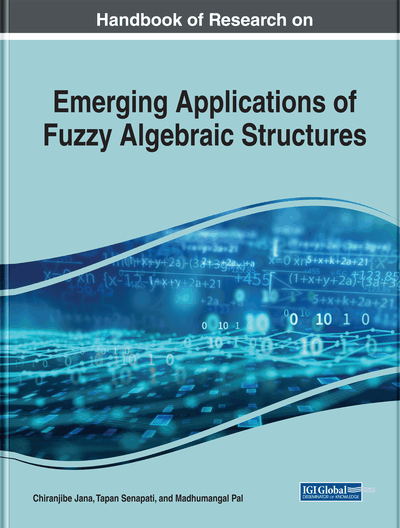Interval-Valued Fuzzy H-Ideals on ß-Algebra

Prakasam Muralikrishna (Muthurangam Government Arts College, India), Tapan Senapati (Southwest University, China) and Perumal Hemavathi (Sri Venkateswara College of Engineering and Technology, India)
DOI: 10.4018/978-1-7998-0190-0.ch015
Available
\$37.50
No Current Special Offers

Abstract

The notion of interval valued fuzzy set was first introduced by Zadeh as a generalization of fuzzy sets. Using interval valued fuzzy set, various algebraic structures and related topics were discussed. This chapter extends fuzzy H-ideal into interval valued fuzzy H-ideals of β-algebra and deals some related results. It also provides the study on homomorphic images of an interval valued fuzzy H-ideals of β-algebra and the idea of Cartesian product of interval valued fuzzy H-ideals of β-algebra.
Chapter Preview
Top

1. Introduction

Neggers (Neggers, 2002a) and Kim (Neggers, & Kim., 2002) initiated the class of B-algebras which is extended from different classes of algebras such as BCH/BCI/BCK-algebras. They also proposed the notion of β – algebras in which two operations were used. Young Bae Jun et al. (Jun, & Kim., 2012) are also explored the notion of β – algebras and related topics.

Abu Ayub Ansari et al. (Ansari, & Chandramouleeswaran., 2013),((Ansari, & Chandramouleeswaran., 2014),(Ansari, & Chandramouleeswaran., 2014a) defined the notion of fuzzy β – subalgebras of β – algebras and inverstigated some of their properties. Further, the authors extended their new ideas towards fuzzy β – ideals of β – algebras. By introducing the concepts of Cartesian product of fuzzy β – ideals of β – algebras. In 2014 the authors of (Ansari, & Chandramouleeswaran., 2014b) introduced the notion of T-fuzzy of β – subalgebras of β – algebras.

After Zadeh’s (Zadeh, 1965) presentation of fuzzy sets, there have been various speculations of this crucial idea. In (Zadeh, 1975) likewise the authors presented the idea of interval valued fuzzy subsets where the estimations of the membership functions are intervals of numbers rather than the numbers.

Biswas and Rosenfeld (Biswas, & Rosenfeld., 1994) proposed the notion of fuzzy subgroups with interval valued membership functions. Fuzzy BCI-subalgebras with interval valued membership functions was studied by Sung Min Houng et al. (Hong, Jun, Kim, & Kim., 1993). Further the new idea of interval valued fuzzy subalgebras/ideals in BCK-algebras was propounded by Jun (Jun, 2000) and also the author (Young, & Min., 2001) redefined the concept of fuzzy subalgebras of BCK/BCI-algebras.

Khalid et al. (Khalid, & Ahmad., 1999) introduced the notion of Fuzzy H-ideals in BCI-algebras. The concept of intuitionistic L-fuzzy H-ideals using BF-algebras has been presented by Muralikrishna et al. (Muralikrishna, & Chandramouleeswaran., 2011). In 2017, Sabarinathan et al. (Sabarinathan, Muralikrishna, & Kumar., 2017) studied the concepts of Bipolar interval valued fuzzy H-ideals of BF-algebras. The new thought of fuzzy H-ideals in BCK-algebras was proposed by Zhan et al. (Zhan, & Tan., 2005).

In 2015, Rajam et al. (Rajam, & Chandramouleeswaran., 2015) proposed the concept of L-fuzzy T-ideals of β – algebras. Sujatha et al. (Sujatha, Chandramouleeswaran, & Muralikrishna., 2014) opened the notion of product on intuitionistic fuzzy β – subalgebras of β – algebras. Kordi et al. (Kordi, Moussavi, & Ahmad., 2008) defined the notion of fuzzy H-ideals of BCI-algebras with interval valued membership functions. Recently, the concept of interval valued fuzzy β – subalgebras were initiated by Hemavathi et al.(Hemavathi, Muralikrishna, Palanivel., 2015). In 2017 (Hemavathi, Muralikrishna, Palanivel., 2017) the authors extended the notion of interval valued fuzzy β – ideals of β – algebras. With all these ideas, in this chapter, we discuss the ideas of interval valued fuzzy H-ideals on β – algebras.

This work entitled as “Interval valued fuzzy H-ideals on β-algebras which is divided into four subsections as follows:

Complete Chapter List

Search this Book:
Reset